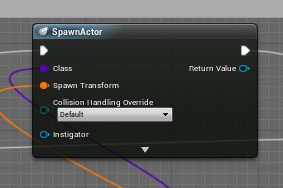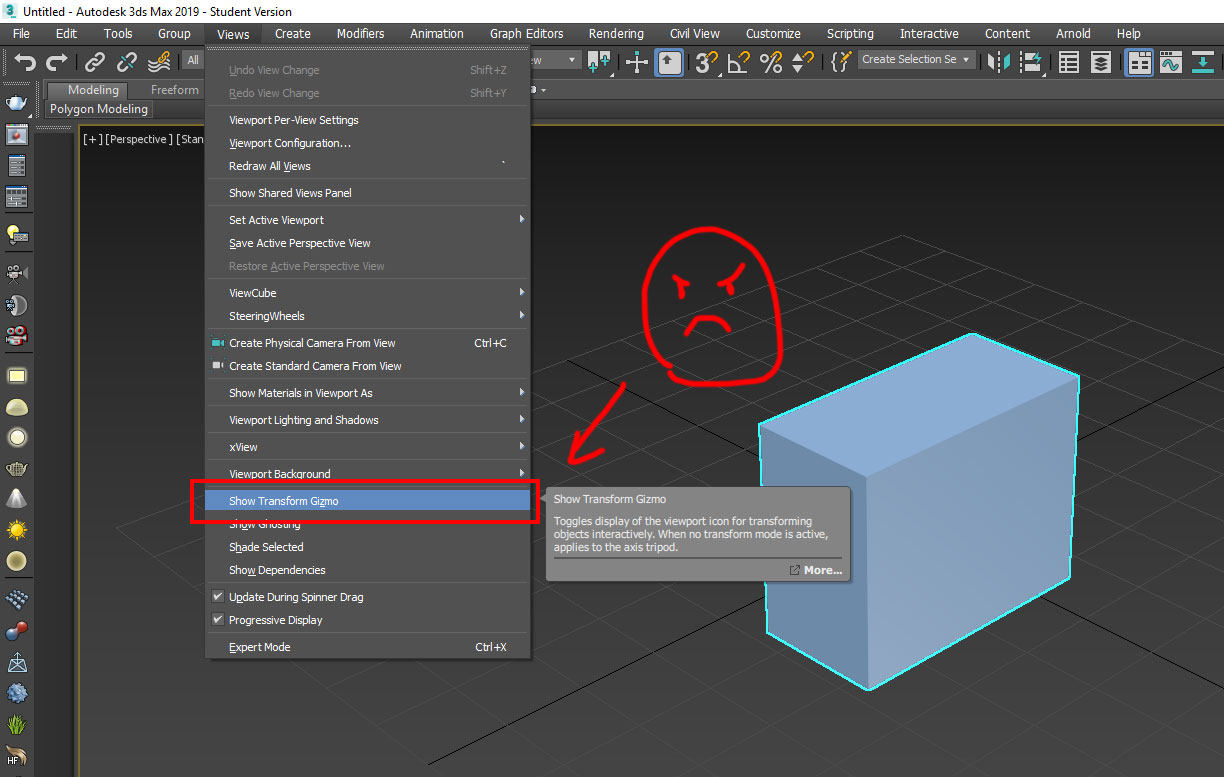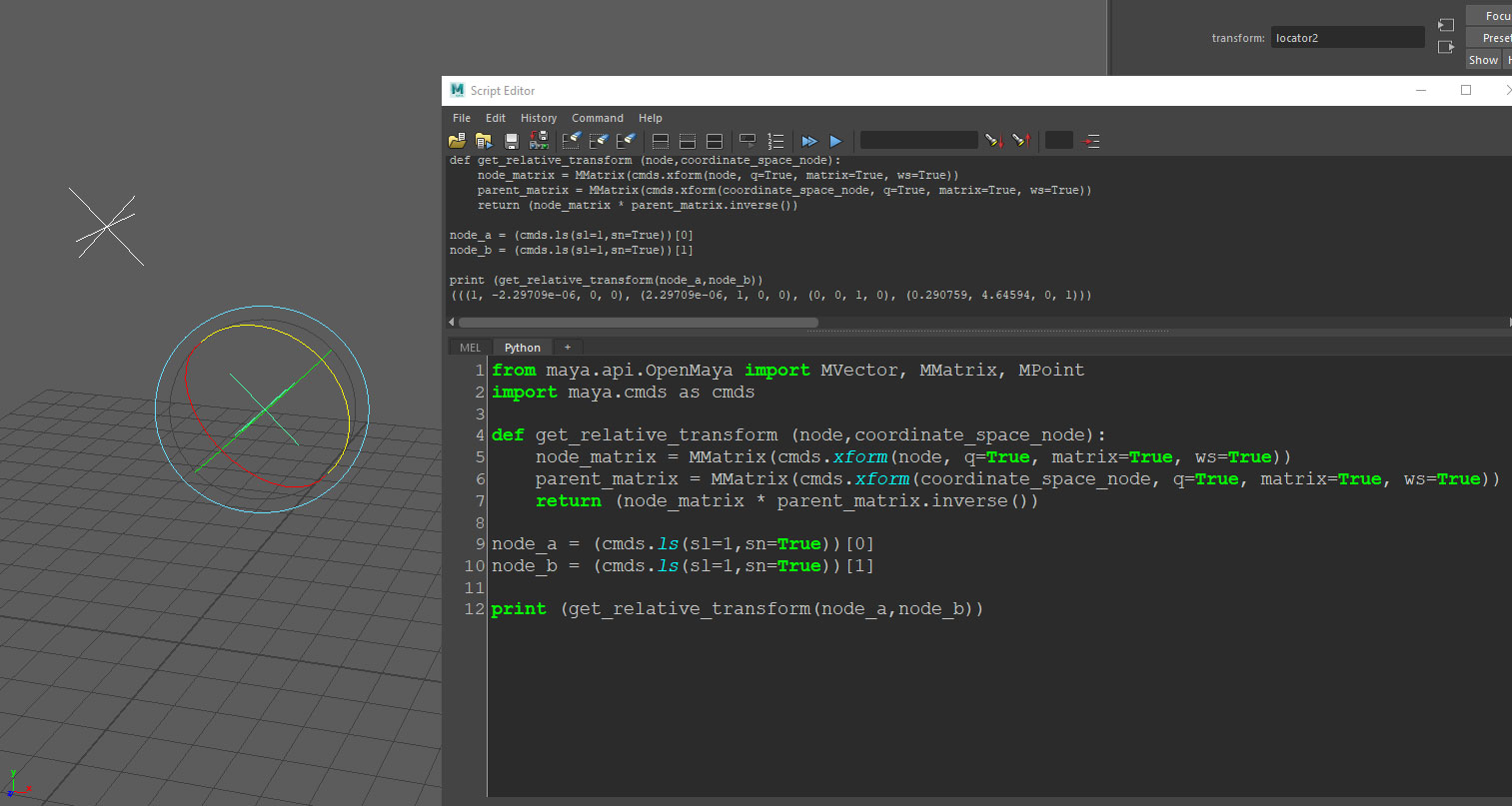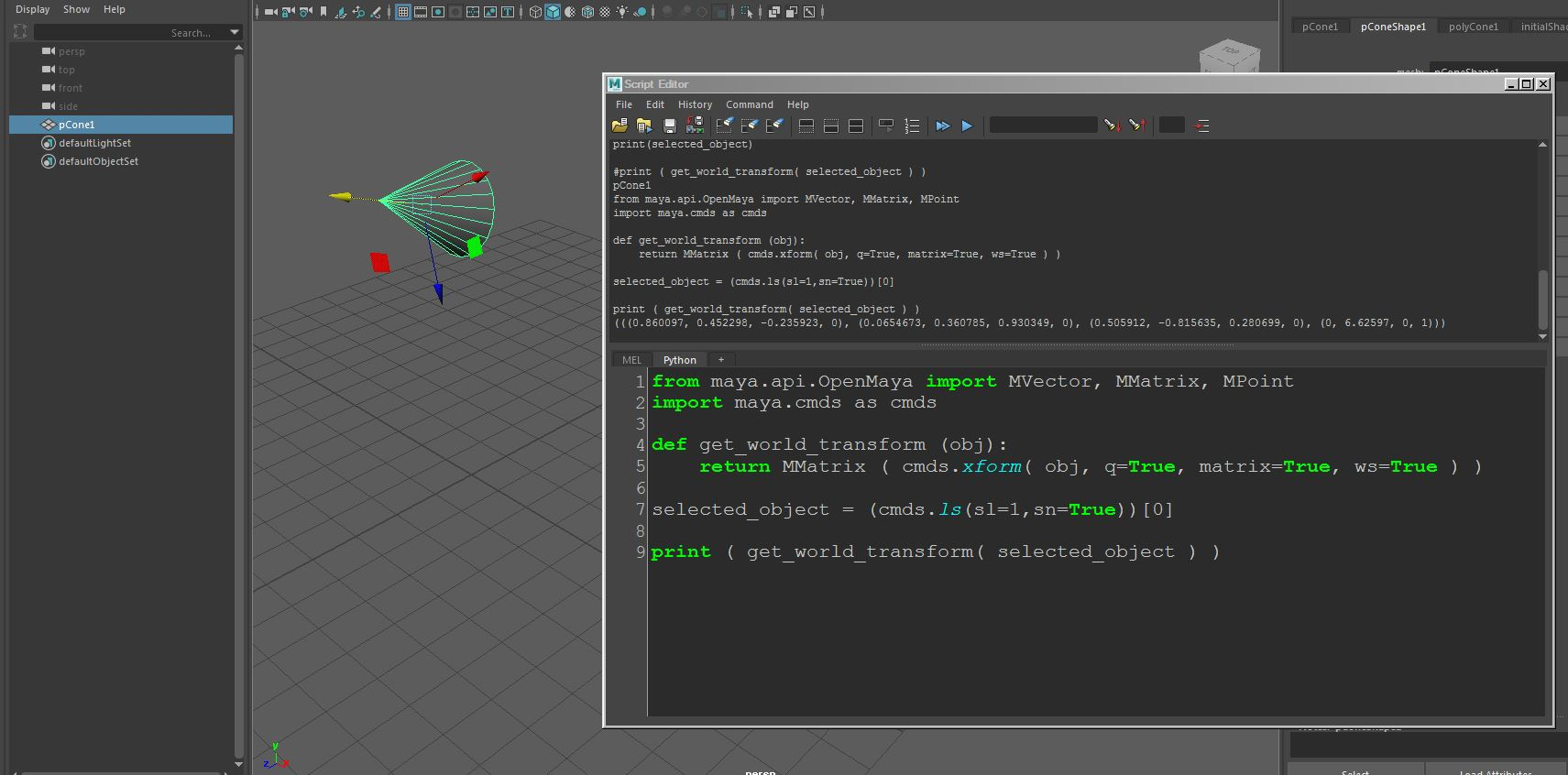## UE4 Blueprints – Spawn Actor Transform Note..

Software:
Unreal Engine 4.24

Short version:
When Spawning new actors via the SpanActor Blueprint node, initial transform must be supplied to the SpanActor node, and not defined in the spawned Actor Class’s Blueprint.Explanation:
Just found out the hard way, that when you spawn an Actor using the SpawnActor blueprint node, the transform data connected to the SpawnActor node is actually applied after the actors Construction Script and BeginPlay Event.
This means any transform you will try to apply in the spawned Actor’s Blueprint will be overridden and therefore not work.

## UE4 – Python – Placing level actors bottom at Z 0.0

Software:
Unreal Engine 4.22

This simple Unreal Editor Python example sets the Z axis location of all actors with names beginning with ‘Sphere_’ in a way that their bottom (minimum Z bound) is at height 0.0.

> learn how to run Python scripts in the UE4 Editor

```import unreal
from unreal import Vector

lst_actors = unreal.EditorLevelLibrary.get_all_level_actors()
print('place actors at 0 z')
for act in lst_actors:
act_label = act.get_actor_label()
if 'Sphere_' in act_label:
print('placing: {}'.format(act_label))
act_location = act.get_actor_location()
act_bounds = act.get_actor_bounds(False)
act_min_z = act_bounds.z - act_bounds.z
location_offset = Vector(act_location.x, act_location.y, act_location.z - act_min_z)
act.set_actor_location(location_offset, False, False)```

* note that when copying and pasting a script from this example, the indentation may not be pasted correctly.

Note:
The get_actor_bounds unreal.Actor class method returns a tuple object containing 2 unreal.Vector objects, the first being world space location of the actor geometric center, and the second is the corner of the bounding box relative to the center.

‘Sphere_*’ actors before running the script:‘Sphere_*’ actors after running the script:## 3ds max – Transform Gizmo disappearing

Software:
3ds max 2019

I’ve been operating 3ds max for 20 years and this always gets me by surprise again and again, especially when working with students 🙁

In the past, the option to hide/show the Transform Gizmo had a hot key X, and curiously, even though that way it would happen more frequently that the Gizmo would disappear by mistake, when it was X I actually remembered it, and remembered to tell my students to just press X.

VIews > Show Transform Gizmo

I never remember it,
And also, I can’t logically except that it’s a system setting that is kept even when resetting the scene or opening a new file.## Maya Python scripting – Getting an object’s transform matrix relative to another object’s coordinates

Software:
Maya 2018

How to get an object transformation matrix relative to another object’s coordinates:
* The following script requires selecting 2 objects, the function will return the transform matrix of the first object relative to the transform matrix of the second.

```from maya.api.OpenMaya import MVector, MMatrix, MPoint
import maya.cmds as cmds

def get_relative_transform (node,coordinate_space_node):
node_matrix = MMatrix(cmds.xform(node, q=True, matrix=True, ws=True))
parent_matrix = MMatrix(cmds.xform(coordinate_space_node, q=True, matrix=True, ws=True))
return (node_matrix * parent_matrix.inverse())

node_a = (cmds.ls(sl=1,sn=True))
node_b = (cmds.ls(sl=1,sn=True))

print (get_relative_transform(node_a,node_b))```## Maya Python scripting – Getting an Object’s world-space transform matrix

Software:
Maya 2018

How to get an object transformation matrix in world space coordinates:

```from maya.api.OpenMaya import MVector, MMatrix, MPoint
import maya.cmds as cmds

def get_world_transform (obj):
return MMatrix ( cmds.xform( obj, q=True, matrix=True, ws=True ) )

selected_object = (cmds.ls(sl=1,sn=True))

print ( get_world_transform( selected_object ) )```